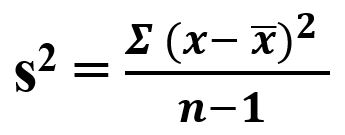# sciPy stats.tvar() function | Python

scipy.stats.tvar(array, limits=None, inclusive=(1, 1)) function calculates the trimmed variance of the array elements along with ignoring the values lying outside the specified limits.

It’s formula –Parameters :
array: Input array or object having the elements to calculate the trimmed variance.
limits: Lower and upper bound of the array to consider, values less than the lower limit or greater than the upper limit will be ignored. If limits is None [default], then all values are used.
inclusive: Decide whether to include the values equal to lower or upper bound, or to exclude them while calculation.

Returns : Trimmed variance of the array elements based on the set parameters.

Code #1:

 `# Trimmed Variance  ` ` `  `from` `scipy ``import` `stats ` `import` `numpy as np  ` ` `  `# array elements ranging from 0 to 19 ` `x ``=` `np.arange(``20``) ` `  `  `print``(``"Trimmed Variance :"``, stats.tvar(x))  ` ` `  ` `  `print``(``"\nTrimmed Variance by setting limit : "``,  ` `      ``stats.tvar(x, (``2``, ``10``))) `

Output:

```Trimmed Variance : 35.0

Trimmed Variance by setting limit :  7.5
```

Code #2: Checking “inclusive” flags

 `# Trimmed Variance  ` ` `  `from` `scipy ``import` `stats ` `import` `numpy as np  ` ` `  `# array elements ranging from 0 to 19 ` `x ``=` `np.arange(``20``) ` ` `  `# Setting limits ` `print``(``"\nTrimmed Variance by setting limit : "``,  ` `      ``stats.tvar(x, (``2``, ``10``)))  ` ` `  `# using flag ` `print``(``"\nTrimmed Variance by setting limit : "``,  ` `      ``stats.tvar(x, (``2``, ``10``), (``False``, ``True``)))  ` ` `  `print``(``"\nTrimmed Variance by setting limit : "``,  ` `      ``stats.tvar(x, (``2``, ``12``), (``True``, ``False``)))  `

Output:

```Trimmed Variance by setting limit :  7.5

Trimmed Variance by setting limit :  6.0

Trimmed Variance by setting limit :  9.16666666667
```

Attention geek! Strengthen your foundations with the Python Programming Foundation Course and learn the basics.

To begin with, your interview preparations Enhance your Data Structures concepts with the Python DS Course.

My Personal Notes arrow_drop_upCheck out this Author's contributed articles.

If you like GeeksforGeeks and would like to contribute, you can also write an article using contribute.geeksforgeeks.org or mail your article to contribute@geeksforgeeks.org. See your article appearing on the GeeksforGeeks main page and help other Geeks.

Please Improve this article if you find anything incorrect by clicking on the "Improve Article" button below.

Article Tags :

Be the First to upvote.

Please write to us at contribute@geeksforgeeks.org to report any issue with the above content.